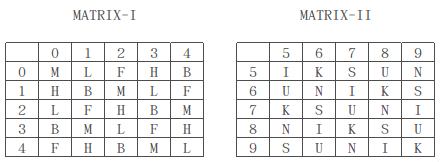# SSC CPO 20 March 2016 Afternoon Shift

Instructions

In the each of the following questions, find the odd word/letters/number pair from the given alternatives.

Instructions

A word is represented by only one set of numbers as given in any one of the alternatives. The set of numbers given in the alternatives are represented by two classes of alphabets as in two matrices given below. The columns and rows of Matrix I are numbered from  0 to 4 and that of Matrix II  are numbered from 5 to 9. A letter from these matrices can be represented first by its row and next by its coloumn, e.g., 'A' can be represented by 01, 20 e.t.c., and 'B' can be represented by 56, 65 e.t.c. Similarly, you have to identify the set for the word given in each question .

Question 16

Question 17

# SILKInstructions

In each of the following questions, select the related word/letters/number from the given alternatives.

Question 18

Question 19

Question 20

OR